### Pay-bill calculation using OOP

Solve the below problem using OPP in C++. Use most suitable OOP architecture to solve this issue.
Problem :
A manufacturing company has classified its executives into four levels for the benefit of certain perks. The level and corresponding perks are shown by Table-1 stated below. An executive’s gross salary includes basic pay, house rent allowance at 25% of basic pay and other perks. Income tax is withheld from the salary on a percentage basis, which is explained in the Table-2 stated below. Write a program that will read and executive’s job number, level number, and basic pay and then compute the net salary after withholding income tax.
Table-1 and Table-2 are attached as picture.

1.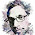#include
using namespace std;
class base
{
public:
int n,bp,lv,hr,result=0,gs=0;
base()
{
cout<<"Input Employee No.: ";
cin>>n;
cout<<"Input Employee Level: ";
cin>>lv;
cout<<"Input Employee Basic Pay: ";
cin>>bp;
hr=bp*25/100;
}
~base()
{

}
};
class appel:public base
{
public:
appel()
{

if(lv==1)
gs=1000+500+bp+hr;

if(lv==2)
gs=750+200+bp+hr;

if(lv==3)
gs=500+100+bp+hr;

if(lv==4)
gs=250+0+bp+hr;
}
void print()
{
if(gs<=2000)
result=gs;
if(gs<=4000&&gs>2000)
result=gs-(gs*3/100);
if(gs<=5000&&gs>4000)
result=gs-(gs*5/100);
if(gs>5000)
result=gs-(gs*8/100);
cout<<endl<<"Employees Gross Payment: "<<result<<endl;
}
~appel()
{

}
};
int main()
{
appel obj2;
obj2.print();
}

2.#include
using namespace std;
class employee
{
public:
int jn,lv,bp,sum=0,net_s=0;
employee(int j, int l, int b);
~employee();
void level_1();
void level_2();
void level_3();
void level_4();
};
employee::employee(int j, int l, int b)
{
jn=j;
lv=l;
bp=b;
}
void employee::level_1()
{
sum=bp+(bp*0.25)+1000+500;
if(sum<=2000)
net_s=sum;
else if(2000>j;
cout<<"Enter level: ";
cin>>l;
cout<<"Enter basic pay: ";
cin>>b;
employee obj(j,l,b);

if(l==1)
obj.level_1();
else if(l==2)
obj.level_2();
else if(l==3)
obj.level_3();
else
obj.level_4();
return 0;
}

3.#include
using namespace std;
class paybill
{
public:

int level,num;
float gp,bp,hr,p,net=0,tax=0;
paybill();
~paybill();
void pay()

{

switch(level)
{
case 1:
p=1000+500;
break ;
case 2:
p=750+200;
break;
case 3:
p=500+100;
break;
case 4:
p=250+0;
break;
default:
cout<<"Eror in acces code:";
}
hr=.25*bp;
gp=bp+hr+p;
if(gp<=2000)
tax=0;
else if(gp<=4000&&gp>2000)
tax=.03*gp;
else if(gp<=5000&&gp>4000)
tax=.05*gp;
else
tax=.08*gp;
net=gp-tax;
cout<<"The level number of employee is :"<>level;
cout<<"Enter number of the employee :";
cin>>num;
cout<<"Enter the basic pay:";
cin>>bp;
}
paybill::~paybill()
{

}

int main()
{

cout<<"it is pay bill calculation using opp :"<<endl<<endl<<endl;
paybill obj;
obj.pay();

}

4.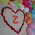#include
using namespace std;

class basic
{
protected:
int job_number, basic_pay, conveyance_allowance, entertainment_allowance;
public:
int level_number;
basic()
{
cout<<"Enter the job number: ";
cin>>job_number;
cout<<"Enter the level number: ";
cin>>level_number;
cout<<"Enter the basic pay: ";
cin>>basic_pay;

if( level_number==1)
{
conveyance_allowance=1000;
entertainment_allowance=500;
}
else if( level_number==2)
{
conveyance_allowance=750;
entertainment_allowance=200;
}
else if( level_number==3)
{
conveyance_allowance=500;
entertainment_allowance=100;
}
else if( level_number==4)
{
conveyance_allowance=250;
entertainment_allowance=0;
}
}
~basic()
{

}
};

class employee: public basic
{
public:
double gross,net_salary,house_rent;
employee()
{
if(basic_pay<=2000)
{
house_rent=basic_pay*25/100;
gross=basic_pay;
}
else if(basic_pay>2000 && basic_pay<=4000)
{
house_rent=basic_pay*25/100;
gross=basic_pay-(3*basic_pay/100);
}
else if(basic_pay>4000 && basic_pay<=5000)
{
house_rent=basic_pay*25/100;
gross=basic_pay-(5*basic_pay/100);
}
else
{
house_rent=basic_pay*25/100;
gross=basic_pay-(8*basic_pay/100);
}

//cout<<endl<<gross;
//cout<<endl<<conveyance_allowance<<endl;
net_salary=gross+house_rent+conveyance_allowance+entertainment_allowance;

cout<<endl<<"Net Salary is: "<<net_salary;
}
~employee()
{

}
};

main()
{
employee obj;
}

5.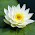using C++

#include
using namespace std;

class Base
{
public:
int numOfEmp;
void takeNumOfEmp(int i);
void printIdLvl(int i);
void empDetails();
protected:
int proNumOfEmp;
double transEmpData;
double basicPay;
};

void Base::takeNumOfEmp(int i)
{
proNumOfEmp = numOfEmp = i;
}
void Base::printIdLvl(int i)
{
if (i == 0)
cout << "Enter Employ Id --: ";
else if (i == 1)
cout << "Enter Employ Level --: ";
}
void Base::empDetails()
{
for (int i = 0; i < numOfEmp; i++){
for (int j = 0; j < 2; j++){
printIdLvl(j);
cin >> transEmpData[i][j];
}
}

for (int i = 0; i < numOfEmp; i++){
cout << "Enter Basic Pay For id " << transEmpData[i] << " --: ";
cin >> basicPay[i];
}
}

class Derive: public Base
{
public:
double perks = {{1000, 500}, {750, 200}, {500, 100}, {250, 0}};
double store;
double home;
void showBasicPay();
void calculateBill();
void showPay();
};

void Derive::showBasicPay()
{
double sum = 0, give;
for (int i = 0; i < proNumOfEmp; i++){
sum = basicPay[i];
give = sum*0.25;
home[i] = give;
store[i] = (sum-give);
}
}

void Derive::calculateBill()
{
for (int i = 0; i < proNumOfEmp; i++){
double total = 0;
if (store[i] <= 2000){
store[i] = store[i];
}
else if (store[i] <= 4000){
total = store[i]*0.03;
store[i] = store[i]-total;
}
else if (store[i] <= 5000){
total = store[i]*0.05;
store[i] = store[i]-total;
}
else if (store[i] > 5000){
total = store[i]*0.08;
store[i] = store[i]-total;
}
}
}

void Derive::showPay()
{
for (int i = 0; i < proNumOfEmp; i++){
int sum = 0;
if (transEmpData[i] == 1){
sum = store[i] + perks + perks + home[i];
store[i] = sum;
}
else if (transEmpData[i] == 2){
sum = store[i] + perks + perks + home[i];
store[i] = sum;
}
else if (transEmpData[i] == 3){
sum = store[i] + perks + perks + home[i];
store[i] = sum;
}
else if (transEmpData[i] == 4){
sum = store[i] + perks + perks + home[i];
store[i] = sum;
}
}
for (int i = 0; i < proNumOfEmp; i++){
cout << "Total payable bill with out TAX for ID " << transEmpData[i] << " --: " << store[i] << endl;
}
}

int main()
{
Derive obj;
int numOfEmp;

cout << "Enter how many employ data do you want to Entry --: "; //******maximum 20 data can be show or store*******
cin >> numOfEmp;

obj.takeNumOfEmp(numOfEmp);
obj.empDetails();
obj.showBasicPay();
obj.calculateBill();
obj.showPay();

return 0;
}

6.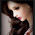ID 201510280
batch 48
#include
using namespace std;
class base
{
public:
int job_number, basic_pay, conveyance_allowance, entertainment_allowance, level_number,house_rent;
basic()
{
cout<<"Enter the job number: ";
cin>>job_number;
cout<<"Enter the level number: ";
cin>>level_number;
cout<<"Enter the basic pay: ";
cin>>basic_pay;
house_rent=basic_pay*25/100;
}
protected:
int total=0;
};
class d:public base
{
public:
float gross,house_rent;
salary()
{
if( level_number==1&&basic_pay<=2000)
{
conveyance_allowance=1000;
entertainment_allowance=500;
gross=basic_pay;
}
else if( level_number==2&&basic_pay>2000&&basic_pay<=4000)
{
conveyance_allowance=750;
entertainment_allowance=200;
gross=basic_pay-(3*basic_pay/100);
}
else if( level_number==3&&basic_pay>4000&&basic_pay<=5000)
{
conveyance_allowance=500;
entertainment_allowance=100;
gross=basic_pay-(5*basic_pay/100);
}
else
{
conveyance_allowance=250;
entertainment_allowance=0;
gross=basic_pay-(8*basic_pay/100);
}
total=gross+house_rent+conveyance_allowance+entertainment_allowance;
cout<<"salary:"<<total;
}
};
int main()
{
d obj;
obj.basic();
obj.salary();
}

7.ID : 201510545
Name : Aubhijit Sarkar

https://www.dropbox.com/s/ufxxkm0pks47reb/main.cpp?dl=0

8.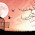//Maisha Fahmida
//Batch:48th
//ID:201511018

#include
using namespace std;

class myclass{
public:
int maisha(int n3, int n4, int n5)
{
n6 = n3;
lev = n4;
n7 = n5;
}
protected:
int n6;
int lev;
int n7;
};

class people : public myclass
{
public:
double sum = 0, sum1 = 0;
double function()
{
if(lev == 1)
{
sum = n7 + (n7 * 0.25) + 500 + 1000;
if (sum <= 2000)
{
sum1 = sum;
cout<<"Enter salary : " < 5000)
{
sum1 = sum - (sum * 0.08);
cout<<"Enter salary : " < 5000)
{
sum1 = sum - ( sum * 0.08);
cout<<"Enter salary : " < 5000)
{
sum1 = sum - (sum * 0.08);
cout<<"Enter salary : " < 5000)
{
sum1 = sum - (sum * 0.08);
cout<<"Enter salary : " <>n;

cout<<"Enter the level: ";
cin>>n1;

cout<<"Enter the basic pay: ";
cin>>n2;

people ob;
ob.maisha(n, n1, n2);
ob.function();

return 0;
}

9.//Maisha Fahmida
//Batch:48th
//ID:201511018
#include
using namespace std;
class myclass{
public:
int maisha(int n3, int n4, int n5)
{
n6 = n3;
lev = n4;
n7 = n5;
}
protected:
int n6;
int lev;
int n7;
};
class people : public myclass
{
public:
double sum = 0, sum1 = 0;
double function()
{
if(lev == 1)
{
sum = n7 + (n7 * 0.25) + 500 + 1000;
if (sum <= 2000)
{
sum1 = sum;
cout<<"Enter salary : " < 5000)
{
sum1 = sum - (sum * 0.08);
cout<<"Enter salary : " < 5000)
{
sum1 = sum - ( sum * 0.08);
cout<<"Enter salary : " < 5000)
{
sum1 = sum - (sum * 0.08);
cout<<"Enter salary : " < 5000)
{
sum1 = sum - (sum * 0.08);
cout<<"Enter salary : " <>n;
cout<<"Enter the level: ";
cin>>n1;
cout<<"Enter the basic pay: ";
cin>>n2;
people ob;
ob.maisha(n, n1, n2);
ob.function();
return 0;
}

Comment Here